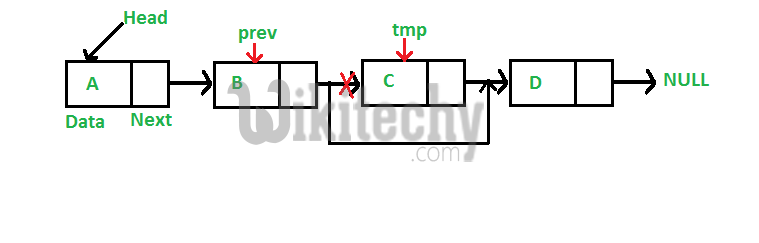# C++ Algorithm – Deleting a node in Linked List

Cp++ Algorithm - Deleting a node in Linked List - Linked List - We have discussed Linked List Introduction and Linked List Insertion in previous

We have discussed Linked List Introduction and Linked List Insertion in previous posts on singly linked list.

Let us formulate the problem statement to understand the deletion process. Given a ‘key’, delete the first occurrence of this key in linked list.
To delete a node from linked list, we need to do following steps.
1) Find previous node of the node to be deleted.
2) Changed next of previous node.
3) Free memory for the node to be deleted.Since every node of linked list is dynamically allocated using malloc() in C, we need to call free() for freeing memory allocated for the node to be deleted.

C++ Programming:

``````// A complete working C program to demonstrate deletion in singly
#include <stdio.h>
#include <stdlib.h>

struct node
{
int data;
struct node *next;
};

/* Given a reference (pointer to pointer) to the head of a list
and an int, inserts a new node on the front of the list. */
void push(struct node** head_ref, int new_data)
{
struct node* new_node = (struct node*) malloc(sizeof(struct node));
new_node->data  = new_data;
}

/* Given a reference (pointer to pointer) to the head of a list
and a key, deletes the first occurrence of key in linked list */
void deleteNode(struct node **head_ref, int key)
{
struct node* temp = *head_ref, *prev;

// If head node itself holds the key to be deleted
if (temp != NULL && temp->data == key)
{
return;
}

// Search for the key to be deleted, keep track of the
// previous node as we need to change 'prev->next'
while (temp != NULL && temp->data != key)
{
prev = temp;
temp = temp->next;
}

// If key was not present in linked list
if (temp == NULL) return;

prev->next = temp->next;

free(temp);  // Free memory
}

// This function prints contents of linked list starting from
// the given node
void printList(struct node *node)
{
while (node != NULL)
{
printf(" %d ", node->data);
node = node->next;
}
}

/* Drier program to test above functions*/
int main()
{

puts("\nLinked List after Deletion of 1: ");
return 0;
}``````

Output:

```Created Linked List:
2  3  1  7
Linked List after Deletion of 1:
2  3  7```